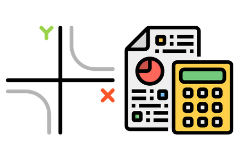## MATHEMATICS DOMAIN#### Further Mathematics 3-4• Study univariate data, bivariate data, financial modelling and more
• Incorporates a practical approach that equips students with essential skills as future citizens
• Suits a student that is not interested in Algebra

Subject Code: V2MFM
Year Level: 12

##### Unit 3 & 4

We complete the core section of the unit. This includes the study of univariate data, bivariate data, recursion and financial modelling and time series.

During the rest of the year you complete two of six optional units offered.

These units are:

Networks (directed and undirected graphs, shortest path, critical path analysis and job allocation).

Matrices (matrix representation of discrete data, matrix arithmetic, solution of simultaneous linear equations for the analysis of problems in practical situations and the calculation of steady state).

The CAS calculator is an essential tool throughout the year, particularly in the core section of the course.

##### What does this mean for me?

Further Mathematics is not the ‘easy option’.

You need to apply yourself and complete all set tasks. You apply your skills with the aid of a CAS calculator. You compile a Rules book which is used in both end of year exams.

##### Assessment
• course work
• two modelling or problem-solving tasks
• one application SAC
• two end of year exams. Exam 1 is multiple choice and Exam 2 is short answer. Calculators and Rules books are allowed in each exam
##### What sort of student would like Further Mathematics?

Someone who:

• Is interested in Maths and who wishes to undertake further study which may have a Mathematics pre-requisite. Many university courses use the data analysis concepts.
• Who is not interested in Algebra.
##### Pre-Requisites

General Mathematics Unit 1 and 2 or Mathematical Methods Unit 1 and 2.

#### Mathematical Methods 3-4• Study Functions and Graphs, Algebra, Calculus, Probability and Statistics
• Can lead to degrees in engineering, sciences, economics, commerce and business
• Must be willing to dedicate time to study outside of class

Subject Code: V2MMM
Year Level: 12

##### Unit 3 & 4

This unit involves the study of Functions and Graphs, Algebra, Calculus, Probability and Statistics. You apply techniques, routines and processes involving rational and real arithmetic, algebraic manipulation, equation solving, graph sketching, differentiation and integration with and without the use of the Ti-nspire calculator, as applicable.

The appropriate use of the Ti-nspire calculator to support and develop the teaching and learning of Mathematics is incorporated throughout the unit. You apply your skills in application problems to model real life situations.

##### Assessment
• coursework
• one technology inclusive exam
• one technology exclusive exam
• one application task of 4-6 hours over 1-2 weeks (Unit 3)
• three modelling / problem solving tasks, each to be 2-3 hours over 1 week (Unit 4)
##### What sort of student would like Mathematical Method Unit 3 & 4?

Someone who:

• Enjoys maths and intends to study or work in areas where having these skills will be an advantage.
• Has a strong interest in Maths and wishes to undertake further study in it and related disciplines.
• Is considering a career in engineering, sciences, economics, commerce and business.

It must not be viewed as merely another pre-requisite for university entrance.

It is designed to promote awareness of the importance of Mathematics in everyday life in a technological society and to promote confidence in making effective use of mathematical ideas, techniques and processes.

##### Pre-Requisites

Mathematical Methods Unit 1 and 2, or its equivalent.

#### Specialist Mathematics 3-4• For students who wish to undertake an in-depth study of mathematics
• Focus on Functions and graphs, Algebra, Calculus, Vectors, Mechanics and Probability and statistics
• Considering a career in engineering? This subject is recommended for you

Subject Code: V2MSM
Year Level: 12

##### Unit 3 & 4

This unit involves many different areas of Mathematics including some familiar ones such as Geometry, Algebra, Trigonometry, Coordinate Geometry, Statistics and Calculus and some unfamiliar ones such as Complex Numbers.

Specialist Mathematics also includes some applied areas of Mathematics such as vectors in two and three dimensions, kinematics and mechanics.

##### What does this mean for me?

You are expected to be able to apply mathematical techniques and processes to the following areas

• rational, real and complex arithmetic, algebraic manipulation
• diagrams and geometric constructions
• equation solving
• graph sketching
• differentiation and integration

You need to apply these mathematical skills both with and without the use of technology.

You also apply your “by hand” skills and use technology to solve problems in routine and unfamiliar contexts.

##### Assessment
• coursework
• modelling or problem-solving task SACS
• one application SAC
• technology inclusive exam
• technology exclusive exam
##### What sort of student would like Specialist Mathematics Unit 3 & 4?

Someone who:

• Has a strong interest in Maths and who wishes to undertake further study in it and related disciplines.
• Is considering careers in engineering should consider Specialist Mathematics even though it is not an essential pre-requisite for many universities.
##### Pre-Requisites

You need to have completed, or be concurrently studying, Mathematical Methods Unit 3 and 4. Those wishing to undertake Specialist Mathematics should complete Specialist Mathematics Unit 1 and 2. However, it is not essential.